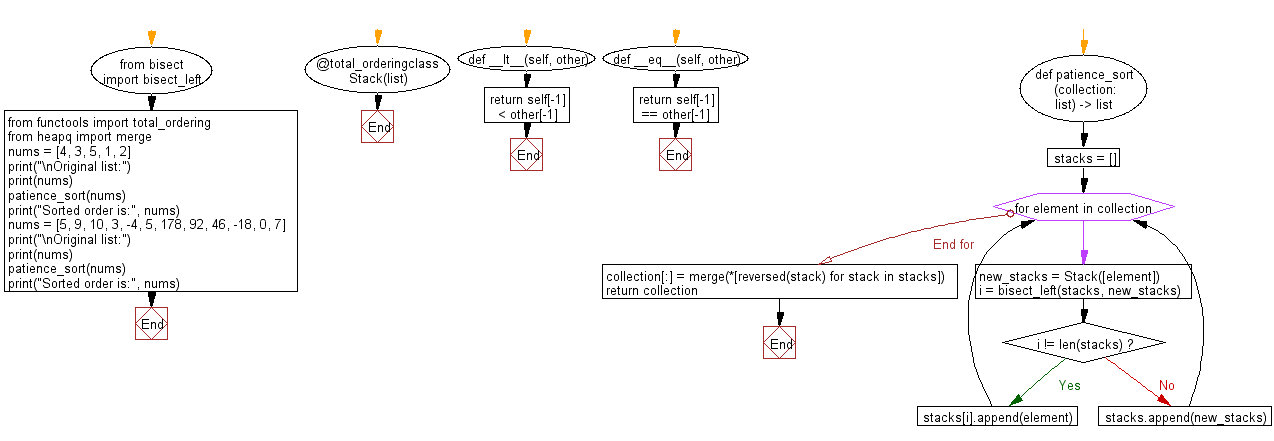﻿ Python: Sort unsorted numbers using Patience sorting - w3resource# Python: Sort unsorted numbers using Patience sorting

## Python Search and Sorting : Exercise-34 with Solution

Write a Python program to sort unsorted numbers using Patience sorting.

From Wikipedia:
In computer science, patience sorting is a sorting algorithm inspired by, and named after, the card game patience. A variant of the algorithm efficiently computes the length of a longest increasing subsequence in a given array.
The algorithm's name derives from a simplified variant of the patience card game. The game begins with a shuffled deck of cards. The cards are dealt one by one into a sequence of piles on the table, according to the following rules.
1. nitially, there are no piles. The first card dealt forms a new pile consisting of the single card.
2. Each subsequent card is placed on the leftmost existing pile whose top card has a value greater than or equal to the new card's value, or to the right of all of the existing piles, thus forming a new pile.
3. When there are no more cards remaining to deal, the game ends.

Sample Solution:

Python Code:

``````#Ref.https://bit.ly/2YiegZB
from bisect import bisect_left
from functools import total_ordering
from heapq import merge
@total_ordering
class Stack(list):
def __lt__(self, other):
return self[-1] < other[-1]
def __eq__(self, other):
return self[-1] == other[-1]
def patience_sort(collection: list) -> list:
stacks = []
# sort into stacks
for element in collection:
new_stacks = Stack([element])
i = bisect_left(stacks, new_stacks)
if i != len(stacks):
stacks[i].append(element)
else:
stacks.append(new_stacks)

# use a heap-based merge to merge stack efficiently
collection[:] = merge(*[reversed(stack) for stack in stacks])
return collection
nums = [4, 3, 5, 1, 2]
print("\nOriginal list:")
print(nums)
patience_sort(nums)
print("Sorted order is:", nums)
nums = [5, 9, 10, 3, -4, 5, 178, 92, 46, -18, 0, 7]
print("\nOriginal list:")
print(nums)
patience_sort(nums)
print("Sorted order is:", nums)
```
```

Sample Output:

```Original list:
[4, 3, 5, 1, 2]
Sorted order is: [1, 2, 3, 4, 5]

Original list:
[5, 9, 10, 3, -4, 5, 178, 92, 46, -18, 0, 7]
Sorted order is: [-18, -4, 0, 3, 5, 5, 7, 9, 10, 46, 92, 178]
```

Flowchart:Python Code Editor:

What is the difficulty level of this exercise?

Test your Programming skills with w3resource's quiz.

﻿

## Python: Tips of the Day

The Zip() Function:

```>>> students = ('John', 'Mary', 'Mike')
>>> ages = (15, 17, 16)
>>> scores = (90, 88, 82, 17, 14)
>>> for student, age, score in zip(students, ages, scores):
...     print(f'{student}, age: {age}, score: {score}')
...
John, age: 15, score: 90
Mary, age: 17, score: 88
Mike, age: 16, score: 82
>>> zipped = zip(students, ages, scores)
>>> a, b, c = zip(*zipped)
>>> print(b)
(15, 17, 16)
```